Next: Continuation for one dimensional Up: Examples Previous: Example 1   Contents

Example 2

Consider the problem of finding the coordinates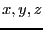of a point that lie on the surface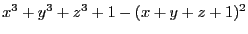, is inside the sphere centered at (-1,-1,0) of radius 1 and is the closest possible to the center of this sphere, with the constraint thatlie in the range [-2,2]. Thus we have: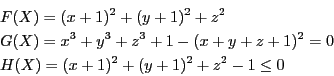With epsilonf=0.0001 we find out that the point is located at at (-0.747,-0.747,0.086059) which is well inside the sphere and that the minimal distance is 0.13529.

We may also compute the minimal distance not to a point but to a line segment, for example defined by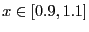,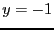,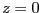. In that case the optimum function in the evaluation procedure may be defined as:

Sqr(x+INTERVAL(0.9,1.1))+Sqr(y+1)+Sqr(z)
and with epsilonf=0.0001 the algorithm will return that the minimal distance lie in the range [0.0907,0.1925].

Jean-Pierre Merlet 2012-12-20